Next: Dielectric Liquids in Electrostatic Up: Electrostatics in Dielectric Media Previous: Force Density Within Dielectric

# Clausius-Mossotti Relation

Let us now investigate what a dielectric equation of state actually looks like. Suppose that a dielectric medium is made up of identical molecules that develop a dipole moment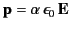(585)

when placed in an electric field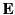. The constantis called the molecular polarizability. If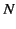is the number density of such molecules then the polarization of the medium is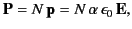(586)

or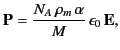(587)

whereis the mass density,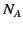is Avogadro's number, and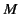is the molecular weight. But, how does the electric field experienced by an individual molecule relate to the average electric field in the medium? This is not a trivial question because we expect the electric field to vary strongly (on atomic lengthscales) within the dielectric.

Suppose that the dielectric is polarized by a mean electric fieldthat is uniform (on macroscopic lengthscales), and directed along the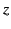-axis. Consider one of the dielectric's constituent molecules. Let us draw a sphere of radius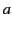around this particular molecule. The surface of the sphere is intended to represent the boundary between the microscopic and the macroscopic ranges of phenomena affecting the molecule. We shall treat the dielectric outside the sphere as a continuous medium, and the dielectric inside the sphere as a collection of polarized molecules. According to Equation (530), there is a bound surface charge of magnitude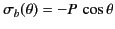(588)

on the inside of the sphere's surface, where,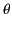,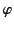are conventional spherical coordinates, and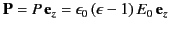is the uniform polarization of the uniform dielectric outside the sphere. The magnitude of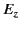at the molecule due to this surface charge is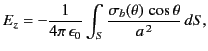(589)

where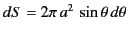is an element of the surface. It follows that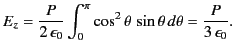(590)

It is easily demonstrated, from symmetry, that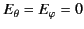at the molecule. Thus, the field at the molecule due to the bound charges distributed on the inside of the sphere's surface is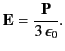(591)

The field due to the individual molecules within the sphere is obtained by summing over the dipole fields of these molecules. The electric field a distancefrom a dipole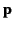is (see Section 2.7)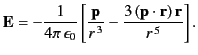(592)

It is assumed that the dipole moments of the molecules within the sphere are all the same, and also that the molecules are evenly distributed throughout the sphere. This being the case, the value ofat the molecule due to all of the other molecules within in the sphere,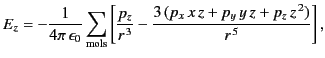(593)

is zero, because, for evenly distributed molecules,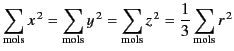(594)

and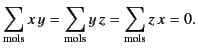(595)

It is also easily demonstrated that. Hence, the electric field at the molecule due to the other molecules within the sphere averages to zero.

It is clear that the net electric field experienced by an individual molecule is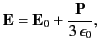(596)

which is larger than the average electric field,, in the dielectric. The above analysis indicates that this effect is ascribable to the long range (rather than the short range) interactions of the molecule with the other molecules in the medium. Making use of Equation (588), as well as the definition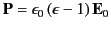, we obtain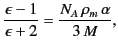(597)

which is known as the Clausius-Mossotti relation. This expression is found to work very well for a wide class of dielectric liquids and gases. The Clausius-Mossotti relation also yields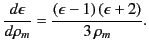(598)Next: Dielectric Liquids in Electrostatic Up: Electrostatics in Dielectric Media Previous: Force Density Within Dielectric
Richard Fitzpatrick 2014-06-27